##### Actions

(diff) ← Older revision | Latest revision (diff) | Newer revision → (diff)

A type of arrangement of the trajectories of an autonomous system of planar ordinary differential equations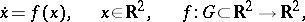(*)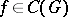, whereis the domain of uniqueness, in a neighbourhood of a singular point (equilibrium position). This type is characterized as follows. There is a neighbourhoodofsuch that for all trajectories of the system that start in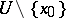, both the positive and negative semi-trajectories are diverging (as time passes they leave any compact set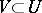). The exceptions are four trajectories, called the separatrices of the saddle. For two of these, the negative semi-trajectories are diverging and the positive semi-trajectories converge to, and for the other two the situation is just the opposite. The first two separatrices are called stable, and the second two, unstable. The stable separatrices, completed by, form a smooth curve through— the stable manifold of the saddle. The unstable separatrices, together with, form the smooth unstable manifold of the saddle. The pointis also called the saddle.

The saddleis unstable in the sense of Lyapunov (cf. Lyapunov stability). Its Poincaré index is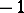(cf. Singular point). For a system (*) of class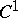(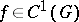) with a non-zero matrix, a stationary pointis a saddle when the eigenvalues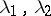ofsatisfy the condition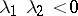(a simple saddle; see Fig. a, where) but it can also be a saddle when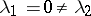, or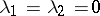.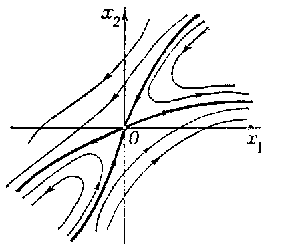Figure: s083020a

In any of these cases the separatrices of the saddle are tangential atto the directions defined by the eigenvectors of.

If the system (*) is linear (, whereis a constant matrix with eigenvaluesand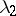), then a pointof it is a saddle only when. In this case the separatrices of the saddleare rectilinear, and all the other trajectories (distinct from) are affine images of the hyperbolas of the form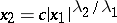,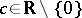(Fig. b).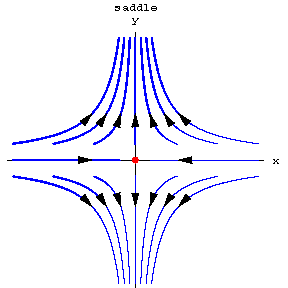Figure: s083020b

The term "saddle" is also used for any of the arrangements of the trajectories of the system (*) in a neighbourhoodof an isolated stationary point, where only a finite number(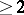) of trajectories in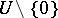approachesand where each of them, completed by, touches at this point in a definite direction (an-separatrix saddle). Certain types of stationary points of autonomous systems of ordinary differential equations of orderare also called saddles. For references, see Singular point of a differential equation.